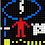## Wednesday, September 26, 2018

### Fun with C - Signed Integer Boundary Conditions

Arithmetic Boundary Condition Miscalculations are very common reasons for security issues in C/C++ applications.

Arithmetic Boundary Conditions:
Integer is one of the most important datatype in C. It has minimum and maximum possible values determined by underlying representation in memory.

Following table shows typical size and min/max range representation for Integer types.

OS - Ubuntu 18.04 ( 32 Bit )
OS - CentOS 7 ( 64 Bit)
"What happens if .....?"
Here,  very basic question - what happens if some operation attempts to cross mentioned Integer type boundary ? In this case, the result of simple arithmetic operations cannot be stored in variable as it is in resulting representation.

For our discussion, we are only concerned about "signed" numbers. As per above integer type table, maximum positive value hold by "int" or "signed integer"  is decimal 2147483647 i.e. 0x7FFFFFFF. If we add 1 into this number the result is 0x80000000 which is a maximum negative number accepted by "signed integer" i.e. -2147483648. In short, large positive number plus small positive number resulted into large negative number and vice versa for negative values.

`````` (gdb) list
1     void main()
2     {
3          int a ;
4          a = 0x7FFFFFFF;
5          a = a + 0x01;
6
7     }
(gdb) s
5          a = a + 0x01;
(gdb) x &a
0xbffff534:     0x7fffffff
(gdb) s
7     }
(gdb) x &a
0xbffff534:     0x80000000
(gdb) print /d a
\$1 = -2147483648
(gdb)
``````

We can see that when operation results crossed maximum positive integer value, number is converted into negative value. Similarly, as operation can overflow boundary of signed positive number , some operations can also result into underflow issues.

Impact:
Boundary overflow and type conversion related subtle issues cause major security impact on resource sensitive operations such as memory management. Due to value wrapping, we can trick program to assign additional memory chunk than what is expected. In short , we can influence program's memory management routines.

For example -
`````` len = packet_read_field(sfd) ;
In above example , consider read_data works similar to how read(2) works. If user craft  packet with negative value into specific field, then value of "signed length" variable will be negative. Now when this value is used to read data , this negative value is passed into read_data() function which expects 3rd argument "len" to be size_t i.e. unsigned integer value.  In this case type conversion operation takes place and  negative value of "len" is converted into positive unsigned integer and passed to read_data() function.  End result, program will read huge number of data from input and place it into buffer. This will lead to overflow and unexpected security exposures.

Example Vulnerability:

CVE-2018-14634 - Mutagen Astronomy: Integer overflow in Linux's create_elf_tables()

Recently, Qualys released security advisory for "Integer Overflow" issue in create_elf_tables() function.
Let's analyze the vulnerable function create_elf_tables() in binfmt_elf.c
``````  150 #define STACK_ROUND(sp, items) \
151     (((unsigned long) (sp - items)) &~ 15UL)
...
165 create_elf_tables(struct linux_binprm *bprm, struct elfhdr *exec,
...
169     int argc = bprm->argc;
170     int envc = bprm->envc;
...
178     int items;
...
190     p = arch_align_stack(p);
...
287     items = (argc + 1) + (envc + 1) + 1;
288     bprm->p = STACK_ROUND(sp, items);
...
295     sp = (elf_addr_t __user *)bprm->p;  ``````

It line 287 code performs some arithmetic operation -
``items = (argc + 1) + (envc + 1) + 1;  ``
where -
argc:
- Part of "linux_binprm" structure, this structure is used to hold the arguments that are used when loading binaries.
- It represents - Maximum number of argument strings passed to execve()
- Which is defined as #define MAX_ARG_STRINGS 0x7FFFFFFF
envc:
- Part of "linux_binprm" structure, this structure is used to hold the arguments that are used when loading binaries.
- It represents - Maximum number of environment variable strings passed to execve()
- It is defined as -
bprm->envc = count(envp, MAX_ARG_STRINGS);
#define MAX_ARG_STRINGS 0x7FFFFFFF

The good news is - "argc" and "envc" both values can be controlled. So for exploitation we need to craft huge "argc" and "envc" values and overflow "signed - items" value , which will then becomes negative. This value is later used for some stack related operations , so gives control over stack manipulation. This control is very useful in later phase of exploitation.
``````  150 #define STACK_ROUND(sp, items) \
151     (((unsigned long) (sp - items)) &~ 15UL)  ``````
1.1.